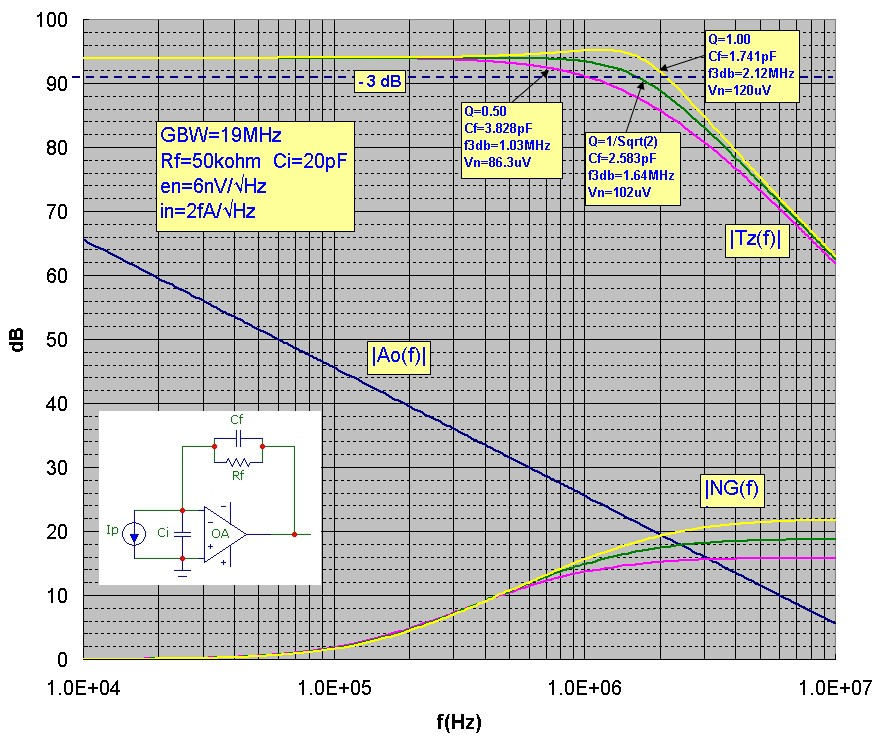# Transimpedance Reference Data

May 01, 2012

The design simulation curves below show transimpedance and noise gain plots for a basic transimpedance circuit with the values shown. The op-amp is modelled as ideal except for a finite gain-bandwidth product described by a single-pole roll-off. Plots for the three cases of interest are shown in the first graph corresponding to Q values of 0.500, 1/√2, and 1.00. All parameters are the same for the three plots except the Cf value. Data shown for each plot includes the transimpedance 3dB bandwidth and the total output voltage noise (integrated over all frequency) calculated exactly for the model used. This reference data can be useful in checking the accuracy of simulation software, or evaluating the importance of real op-amp characteristics or other effects not included here. The second set of graphs shows transimpedance and noise-gain plots for a wider range of Cf values:References: